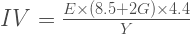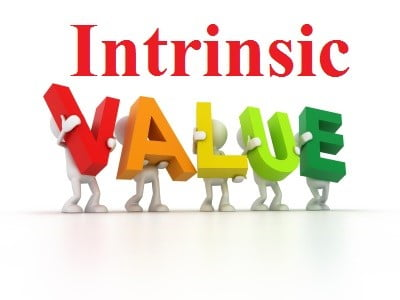View : 369 Click : 0November 5, 2015

# Benjamin Graham Instrinsic value formula for stock valuation

November 5, 2015

There are many ways and formulas to calculate the approximate intrinsic value of any stock. The simplest one is the Benjamin Graham intrinsic value formula. Benjamin Graham success story inspires the investors, he  is know as the father of fundamental analysis

Intrinsic value is an estimated value. It is a value that reflects the real worth of the company. The assets and earning potential is what that makes the company worth.

When the stock is trading below the intrinsic value it is consider undervalued and when it trades above the intrinsic value it is considered over valued. The stock price tends to settle towards intrinsic value.

Benjamin Graham Basic intrinsic value formula

This basic formula first appeared in the Benjamin Graham’s The Intelligent Investor Book published in 1974 . You can find this formula in the Chapter 11 Security Analysis for the Lay Investor page 295Where

E = earnings per share

G = the anticipated growth rate over a projected period (normally 10 years)

Graham observed that the no growth company or the flat growth company trades at the average PE ratio of 8.5. Therefore he used the number 8.5 in the intrinsic value formula.

Benjamin Graham Modified Intrinsic stock price valuation formulawhere:

IV = Intrinsic value
E= Earnings per share
G= expected growth rate
Y= the current yield of triple A rated corporate bonds

Graham believed that investment in equities is good only when they can give better returns than  bonds. Graham chose the figure of 4.4 because this was the AAA corporate bond rate  at the time.  The current bond rate can change, therefore he introduced the term Y which is the current bond yield of AAA rated corporate bond.

The successful results of this formula depends on the estimate of Growth rate G. Different analysts uses different growth rate in the formula. Some uses 10 year average growth rate, some take 5 year average growth rate, while some takes industry growth estimation for the sector to which the company belongs. It is better to be conservative with the growth numbers.

Benjamin Graham Intrinsic value formula calculation example

Let us take Yes Bank which is currently trading at 760 and having recent earning per share(EPS)  of 46 Rs. The current AAA corporate bond yield is 7.5%

Year201020112012201320142015
Net Profit477.74727.149771,300.681,617.782,005.36
Growth in %575234332424

The average 5 year growth will be (57+52+34+33+24+24)/5 = 44 %

The growth of private sector banks is  16%

Analysts estimate of growth is 22% in the  FY17

44% growth rate is difficult to achieve in future and the trend is showing the signs of slowdown in growth. Let us go with the private sector growth rate estimate of 16% and punch the values in the formula

IV =  {46 x (8.5 + 2 x 16) x 4.4 }/ 7.5

Intrinsic value  =  1092

Lets assume now that things won’t be easy and Yes bank will grow at 12%, then

Intrinsic value comes at  877 Rs

So higher the estimated growth rate higher will be the intrinsic value.Limitations of Intrinsic value formula

This formula depends a lot on the estimate of growth. There is a no sure way to estimate the growth. Past performance does not dictate future performance. Also there are many factors which impact the stock price. Even with good growth in the net profit the stock may not appreciate much because of other factors like rising debt, reducing margins, increasing NPA, cash flow etc.  Therefore it is always good to consider other factors also.

Benjamin Graham also says to use it with caution. Page 585 of the book The Intelligent Investor says

” We do not suggest that the formula gives the true value of a growth stock, but only that it approximate the results of the more elaborate calculations in vogue. “

The margin of safety

There are always chances that the company can fail to deliver your estimated growth. You can suffer loss when the company doesn’t performance as you expected. Benjamin Graham invented the margin of safety concept that gives more safety to your investment. Warren Buffet is also a great follower of this margin of safety concept. The margin of safety simply means to buy the stock at the price below its intrinsic value, so that if your calculation is wrong you will suffer minimum loss. If you estimate the intrinsic value of some company at 100 then you should buy it below 100.  The margin of safety depends on the risk of business of your selected company. If risk is more your margin of safety should be more.

This site uses Akismet to reduce spam. Learn how your comment data is processed.